### Home > A2C > Chapter 5 > Lesson 5.2.1 > Problem5-67

5-67.
1. Factor each expression in parts (a) and (b). Then, in parts (c) and (d), factor and reduce each expression. Homework Help ✎

1. bx + ax

2. x + ax

3.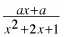4.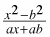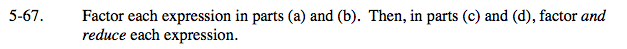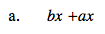x(b + a)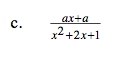$\frac{\textit{a}(\textit{x}+1)}{(\textit{x}+1)^{2}}$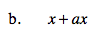x(1 + a)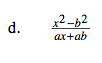$\frac{(\textit{x}+\textit{b})(\textit{x}-\textit{b})}{\textit{a}(\textit{x}+\textit{b})}$